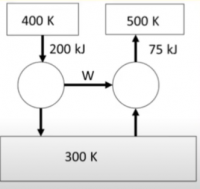LearnChemE

#### Carnot Cycle: Example Problem

Try to solve these problems before watching the solutions in the screencasts.

##### Example Problem 1

A heat pump is used to transfer heat from a reservoir (TC = 250 K) to a higher-temperature reservoir (TH = 300 K). The work done on the pump is 500 J.
1. What is the entropy change for the cycle?
2. Calculate values of QH and QC
3. Determine the coefficient of performance.

##### Example Problem 2

An example to determine if two Carnot cycles, a heat engine and a heat pump, can be combined to transfer heat from 400 K to 500 K and 300 K.